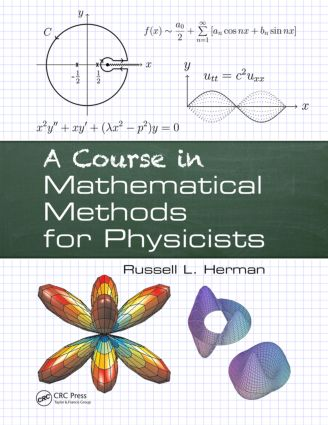# A Course in Mathematical Methods for Physicists

## 1st Edition

CRC Press

776 pages | 420 B/W Illus.

##### Purchasing Options:\$ = USD
Paperback: 9781466584679
pub: 2013-12-04
SAVE ~\$23.00
\$115.00
\$92.00
x
Hardback: 9781138442085
pub: 2017-07-27
SAVE ~\$41.00
\$205.00
\$164.00
x
eBook (VitalSource) : 9780429088919
pub: 2013-12-04
from \$55.00

FREE Standard Shipping!

### Description

Based on the author’s junior-level undergraduate course, this introductory textbook is designed for a course in mathematical physics. Focusing on the physics of oscillations and waves, A Course in Mathematical Methods for Physicists helps students understand the mathematical techniques needed for their future studies in physics. It takes a bottom-up approach that emphasizes physical applications of the mathematics.

The book offers:

• A quick review of mathematical prerequisites, proceeding to applications of differential equations and linear algebra
• Classroom-tested explanations of complex and Fourier analysis for trigonometric and special functions
• Coverage of vector analysis and curvilinear coordinates for solving higher dimensional problems
• Sections on nonlinear dynamics, variational calculus, numerical solutions of differential equations, and Green's functions

### Reviews

"… a welcome and refreshing addition to a rich body of literature. … should fit into the sophomore or junior year of a typical physics undergraduate curriculum. … Engineers and chemistry majors, too, would benefit from taking such an intermediate-level course, perhaps even more so than from a higher-level one. Our own department at Howard University is considering a mid-level math methods course, and I would definitely recommend this textbook as well suited. A Course in Mathematical Methods for Physicists includes plenty of interesting worked-out examples, many of them quite realistic, and uses them to introduce concepts in a reasonable progression. …

Although the subject of mathematical methods has inspired many valuable texts, Herman’s approach, motivated by the physics applications, is novel, seldom used by other authors. The myriad well-chosen worked-out examples and other strengths have earned my firm endorsement …"

—Tristan Hübsch, Howard University, Washington, District of Columbia, USA, from Physics Today

### Table of Contents

Introduction and Review

What Do I Need To Know From Calculus?

What I Need From My Intro Physics Class?

Technology and Tables

Appendix: Dimensional Analysis

Problems

Free Fall and Harmonic Oscillators

Free Fall

First Order Differential Equations

The Simple Harmonic Oscillator

Second Order Linear Differential Equations

LRC Circuits

Damped Oscillations

Forced Systems

Cauchy-Euler Equations

Numerical Solutions of ODEs

Numerical Applications

Linear Systems

Problems

Linear Algebra

Finite Dimensional Vector Spaces

Linear Transformations

Eigenvalue Problems

Matrix Formulation of Planar Systems

Applications

Appendix: Diagonalization and Linear Systems

Problems

Nonlinear Dynamics

Introduction

The Logistic Equation

Autonomous First Order Equations

Bifurcations for First Order Equations

Nonlinear Pendulum

The Stability of Fixed Points in Nonlinear Systems

Nonlinear Population Models

Limit Cycles

Nonautonomous Nonlinear Systems

Exact Solutions Using Elliptic Functions

Problems

The Harmonics of Vibrating Strings

Harmonics and Vibrations

Boundary Value Problems

Partial Differential Equations

The 1D Heat Equation

The 1D Wave Equation

Introduction to Fourier Series

Fourier Trigonometric Series

Fourier Series Over Other Intervals

Sine and Cosine Series

Solution of the Heat Equation

Finite Length Strings

The Gibbs Phenomenon

Green’s Functions for 1D Partial Differential Equations

Derivation of Generic 1D Equations

Problems

Non-sinusoidal Harmonics and Special Functions

Function Spaces

Classical Orthogonal Polynomials

Fourier-Legendre Series

Gamma Function

Fourier-Bessel Series

Sturm-Liouville Eigenvalue Problems

Nonhomogeneous Boundary Value Problems - Green’s Functions

Appendix: The Least Squares Approximation

Appendix: The Fredholm Alternative Theorem

Problems

Complex Representations of Functions

Complex Representations of Waves

Complex Numbers

Complex Valued Functions

Complex Differentiation

Complex Integration

Problems

Transform Techniques in Physics

Introduction

Complex Exponential Fourier Series

Exponential Fourier Transform

The Dirac Delta Function

Properties of the Fourier Transform

The Convolution Operation

The Laplace Transform

Applications of Laplace Transforms

The Convolution Theorem

The Inverse Laplace Transform

Transforms and Partial Differential Equations

Problems

Vector Analysis and EM Waves

Vector Analysis

Electromagnetic Waves

Curvilinear Coordinates

Tensors

Problems

Extrema and Variational Calculus

Stationary and Extreme Values of Functions

The Calculus of Variations

Hamilton’s Principle

Geodesics

Problems

Problems in Higher Dimensions

Vibrations of Rectangular Membranes

Vibrations of a Kettle Drum

Laplace’s Equation in 2D

Three Dimensional Cake Baking

Laplace’s Equation and Spherical Symmetry

Schrödinger Equation in Spherical Coordinates

Solution of the 3D Poisson Equation

Green’s Functions for Partial Differential Equations

Problems

Review of Sequences and Infinite Series

Sequences of Real Numbers

Convergence of Sequences

Limit Theorems

Infinite Series

Convergence Tests

Sequences of Functions

Infinite Series of Functions

Special Series Expansions

The Order of Sequences and Functions

Problems

### About the Author

#### Russell L. Herman

Wilmington, NC, USA

Learn more about Russell L. Herman >>

Russell L. Herman

### Subject Categories

##### BISAC Subject Codes/Headings:
MAT003000
MATHEMATICS / Applied
MAT004000
MATHEMATICS / Arithmetic
SCI040000
SCIENCE / Mathematical Physics
SCI055000
SCIENCE / Physics